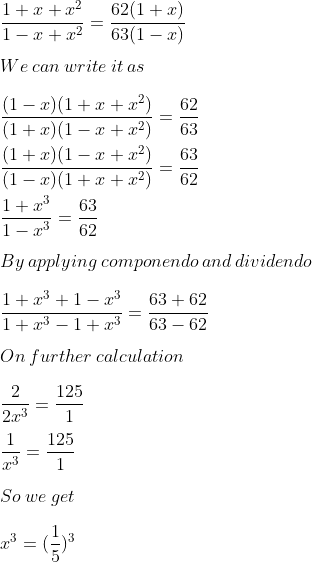Guru

# Solve (1+x+x2) /(1−x+x2) =62(1+x)/63(1−x)

• 0

exam oriented an important question from ML aggarwal, class 10th, chapter 7, ratio and proportion, Avichal publication

In this ques, we have been given an equation with variable x. And we havto solve the equation for x.

Ques 12, 7.3

Share